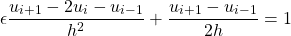## Reproducing a transport instability in convection-diffusion equation

Drawing from Larson-Bengzon FEM book, I wanted to experiment with transport instabilities. It looks there might be an instability in my ocean-ice model but before being able to address that, I wanted to wrap my head around the 1D simplest example one could find. And that is the Convection-Diffusion Equation:

(1)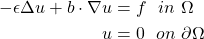The first term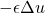is responsible of the smearing of the velocity field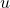proportionally to, and is thus called the diffusion term. Intuitively, it controls how much the neighbors of a given pointare influenced by the behavior of; how much velocities (or temperatures: think of whatever you want!) diffuse in the domain.

The second term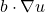controls how much the velocitiesare transported in the direction of the vector field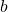, and is thus called the convective term. A requirement for this problem to be well-posed is that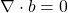— otherwise it would mean that we allow velocities to vanish or to come into existence.

The instability is particularly likely to happen close to a boundary where a Dirichlet boundary condition is enforced. The problem is not localized only close to the boundary though, as fluctuations can travel throughout the whole domain and lead the whole solution astray.

## Transport instability in 1D

The simplest convection-diffusion equation in 1D has the following form:

(2)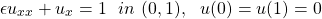whose solution, for smallis approximately just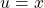. This goes well with the boundary condition at 0, but not with the condition at 1, where the solution needs to go down to 0 quite abruptly to satisfy the boundary condition.

It’s easy to simulate the scenario with FEniCS and get this result (with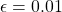and the unit interval divided in 10 nodes):in which we can infer two different trends: one with odd points and one with even points! In fact, if we discretize the 1D equation with finite elements we obtain:

(3)